# A Survey of Question Answering for Math and Science Problem

Overview of the ways to make a machine smart enough to pass the standardized math and science test.

This paper provides a new perspective on thinking about artificial intelligence. Turing test has been proven to be insufficient in measuring artificial intelligence. So now, the standardized math and science tests have been accepted as a way to measure artificial intelligence. The aim of the authors is to give an overview of the ways to make a machine smart enough to pass the standardized math and science test.

## 1. Introduction

The authors first point out that for humans, we are judged by the answers we give for related questions. But we can’t use the same standard to judge machines. Turing test, proposed by Alan Turing in 1950 , judges the machine by whether it can exhibit indistinguishable conversational behavior. Whereas today, standardized tests in mathematics and science have been proposed by Clark and Etzioni  to be a suitable measure for judging machine intelligence. This paper provides us with a brief introduction on how machines are trained to solve math and science problems.

## 2. Question Answering and the Math/Science domain

Question Answering (QA) is a type of task that requires the machine to generate answers to a question, both in natural languages. In modern QA system, there are two kinds of paradigms. One is text-based QA, which relies on large volumes of text. It first retrieves documents that contain the answer by information retrieval methods, then extract the candidate answers from the text, and proceed to rank the answers. The other is knowledge based QA, in which the semantic representation of the question is created and used to query databases of facts. QA on science and mathematics presents unique challenges, because solving these problems require both the good understanding of the questions and containing the internal representation of the problem. Additionally, it should often carry out symbolic computation . This cannot be easily solved by information retrieval or knowledge based methods.

## 3. Question Answering for Science

For the Science questions of standardized tests, there are mainly three questions: basic fact retrieval, inference and world knowledge, and diagrams. the New York Regents Science Exams (NYSED)  is used for this QA task,

### 3.1 Dataset:

The authors provided a few examples of questions on the test and their solutions.

Basic Questions:

1. Which object is the best conductor of electricity? (A) a wax crayon (B) a plastic spoon (C) a rubber eraser (D) an iron nail
2. The movement of soil by wind or water is called (A) condensation (B) evaporation (C) erosion (D) friction

These question can be solved by IR based QA system.

Simple Inference:

1. Which example describes an organism taking in nutrients? (A) dog burying a bone (B) A girl eating an apple (C) An insect crawling on a leaf (D) A boy planting tomatoes in the garden

This question can be solved by simple inference over known facts to arrive at the answer. The machine should know that eating involves taking in nutrients and an apple contains nutrients.

More Complex World Knowledge:

1. A student riding a bicycle observes that it moves faster on a smooth road than on a rough road. This happens because the smooth road has (A) less gravity (B) more gravity (C) less friction (D) more friction

For this question, the machine should be aware of the deeper knowledge and more advanced linguistic capabilities. Like riding a bicycle means moving it, and infer logically the path.

Diagram:

1. Which letter in the Figure 1 points to the plant structure that takes in water and nutrients?

### 3.2 Model

Various approaches are employed to solve this problem. Here, the authors show us two approaches.

Reference  proposes a method of using Integer Linear Programming (ILP). The QA problem is formulated from semi-structured knowledge into a desirable Support Graph, this finally becomes into ILP. The system is shown in Figure 2.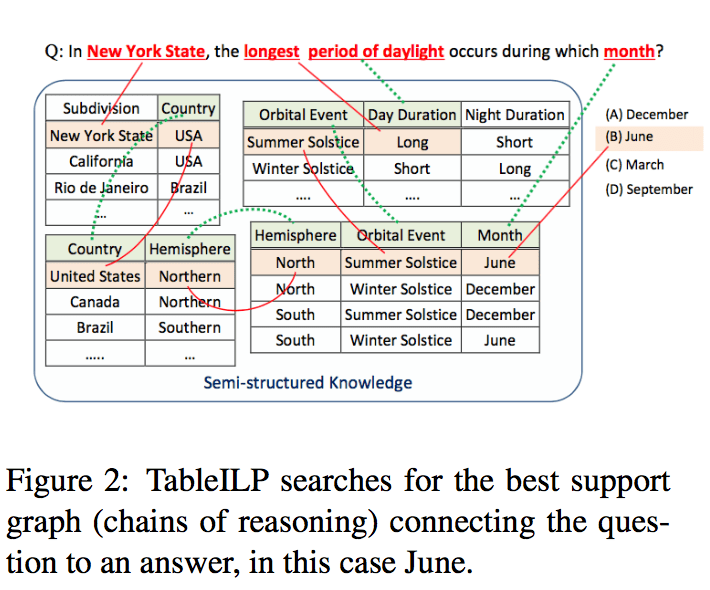Reference  employs an ensemble of solvers to solve the problem at various layers. The layers are shown in Figure 3, the system contains Information Retrieval solver, Pointwise Mutual Information solver, Support Vector Machine solver, RULE solver (which contains hand coded rules), and Integer Linear Programming solver. This system is called ARISTO and it achieved the state-of-the-art.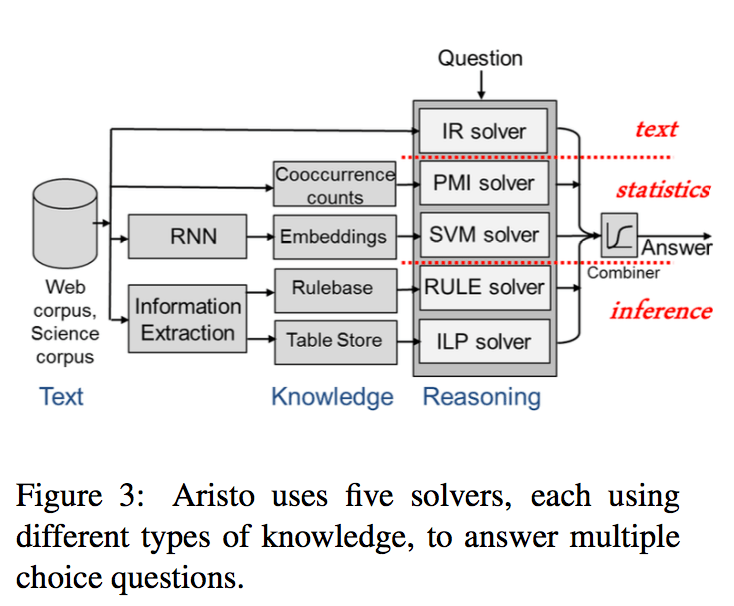## 4. Question Answering for Mathematics

IR systems cannot solve Math questions. To solve mathematical problems, especially arithmetic questions, the system should understand the problem and formulate an equation to calculate. Geometry questions can pose difficulties due to the reliance on diagrams.

### 4.1 Dataset

Similar to part 3.1, the authors provide samples in standardized tests and show the related solutions. Questions from SATs are used for geometry and others are using NYSED.

Algebraic Problems:

1. Molly owns the Wafting Pie Company. This morning, her employees used 816 eggs to bake pumpkin pies. If her employees used a total of 1339 eggs today, how many eggs did they use in the afternoon?
2. Sara’s high school won 5 basketball games this year. They lost 3 games. How many games did they play in all?
3. John has 8 orange balloons, but lost 2 of them. How many orange balloons does John have now?

Language processions are required, and world modeling are also required for the last two.

Geometry Problems
In Figure 4, the machine should understand arithmetic and diagrammatic reasoning.### 4.2 Models

There are four approaches in the paper. One is simple verb categorization that is aimed at solving algebraic word problems. The model is presented in Figure 6, and we can see that the model extract the verbs from the question, and then formulate equations based on verb category.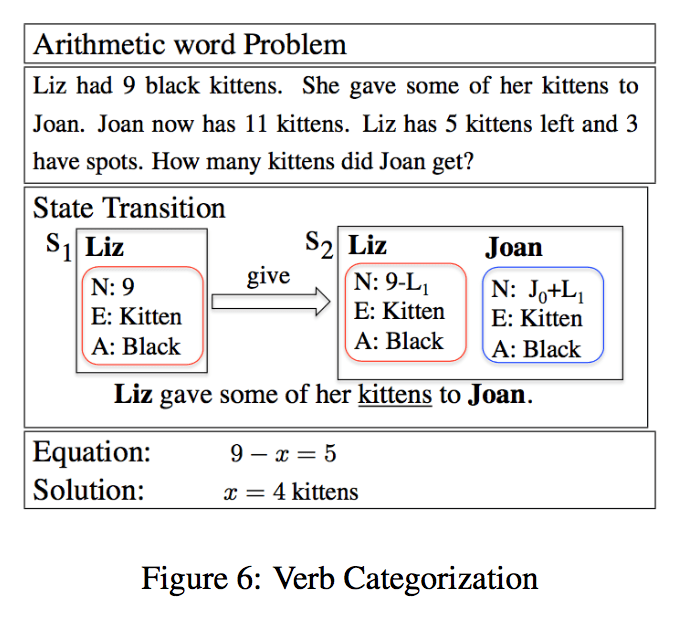Another system is called ALGES, which uses Integer Linear Programming to map the word problems into equation trees, and is more sophisticated. It is shown in Figure 5.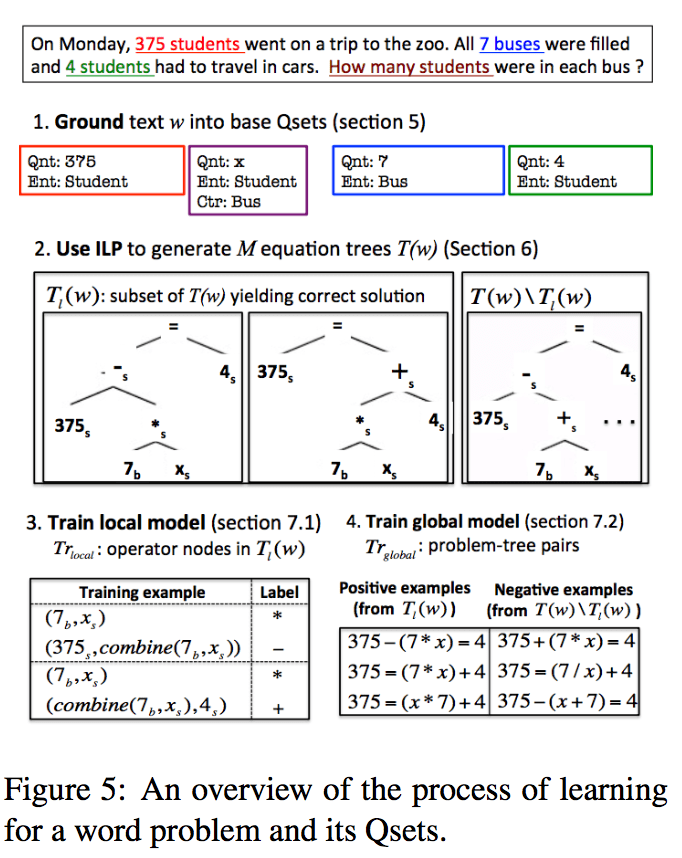The third model is trying to solve geometric diagrams. It is proposed in reference . It uses three steps to align text with geometric diagrams:

1) picking elements that maximize the pixel coverage and identify the primitives in the figure;
2) use agreement between the primitives and textual elements;
3) maximize the coherence of the elements.

In Figure 7 alignment is achieved by the system. Even if it can only achieve the text alignment step out of the three.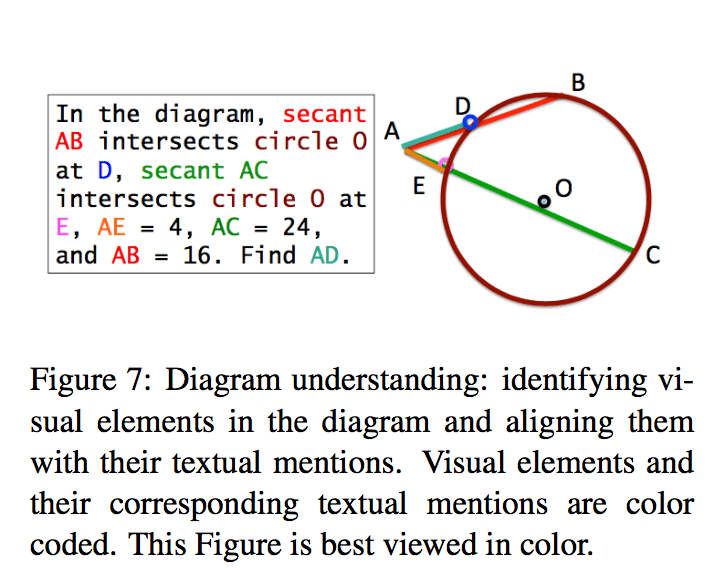The last model is used to solve the geometric diagrams proposed in reference . It follows two steps: 1). use the third model and language processing to convert the diagram and question into logical expressions; 2). use satisfiability solver to deduce the answer. You can see the steps in Figure 8.## 5. Conclusion

The current state of the art cannot solve standardized tests well. For the future, there will be more completed standardized math and science questions. Even if the machine passes the tests, we still can’t say that it’s intelligent. But this will be a landmark event in the history of AI, when it passes Math and Science standardized tests.

## 6. Reference:

 Turing, Alan M. “Computing machinery and intelligence.” Mind 59.236 (1950): 433-460.
 Clark, Peter, and Oren Etzioni. “My Computer Is an Honor Student-but How Intelligent Is It? Standardized Tests as a Measure of AI.” AI Magazine 37.1 (2016): 5-12.
 NYSED. 2014. New York Regents Science Exams. http://www. nysedregents.org/.
 Koncel-Kedziorski, Rik, et al. “Parsing Algebraic Word Problems into Equations.” TACL 3 (2015): 585-597.
 Schoenick, Carissa, et al. “Moving Beyond the Turing Test with the Allen AI Science Challenge.” arXiv preprint arXiv:1604.04315 (2016).
 Seo, Min Joon, et al. “Diagram Understanding in Geometry Questions.” AAAI. 2014.
 Seo, Min Joon, et al. “Solving Geometry Problems: Combining Text and Diagram Interpretation.” EMNLP. 2015.

Author: Shixin Gu | Editor: Joshua Chou